Next: About this document Up: A Really Mean Previous: Background

## Exercises

1. For each of the following functions, verify graphically that the MVT holds and find all values of c, a < c <b, that satisfy the condition1.,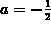, b= 2.
2., a=2, b=5.
3.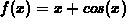,,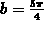.
4.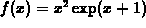, a=-2, b=4.
2. Explain how the MVT applies to support the patrolman's claim.

3. For which values of a and b would the MVT apply to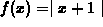?

4. Note that the derivative is not required to be continuous in the MVT. Can you come up with a function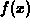that is differentiable, but whose derivative is not continuous? ( Hint - ifis differentiable on an interval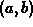, then the derivative cannot have any jump discontinuities. What other kinds of discontinuities are there?)

Sean O Anderson
Wed Sep 27 09:31:40 EDT 1995